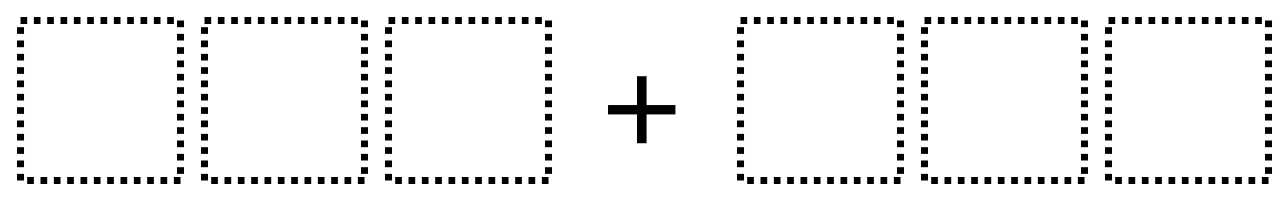Directions: Using the digits 1 to 9 exactly one time each, place a digit in each box two times: once to make a sum that is greater than 700 and once to make a sum that is less than 700. You may reuse all the digits for each sum.### Hint

What place value will be most important to consider? How can you tell whether the sum will be more or less than 700 without adding all of the digits?

There are many possible answers, including 129 + 453 for a sum that is less than 700 and 987 + 654 for a sum that is greater than 700.

Source: Robert Kaplinsky

## Multiply and Divide Within A Hundred 1

Directions: Using the digits 2 to 9 at most one time each, place a digit …

1.Jessie Mommaerts

135 + 246 = 381

678 + 954 = 1,632

2.123 + 456 = 579
486 + 631 = 1117

3.I like openmiddle.com

Thanks,
John Danthuluri

4.111+111=222

222+222=444

444+444=888

888+888=1,776

and that,s it.